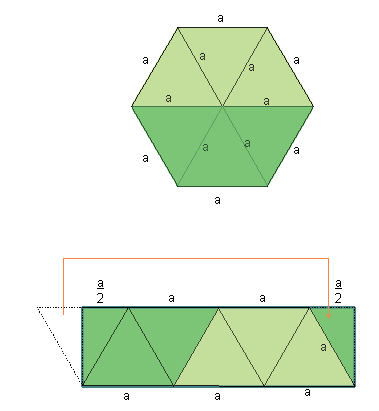Subject: need a formula Name: Scott Who are you: Student please, can you tell me the formula to find the area of a hexagon? if you could it would be greatly appreciated. Hi Scott. Take a look at this diagram of a regular hexagon with side length (and radius) a:The top diagram is the hexagon, and the second diagram shows how I've moved the bottom next to the top, then moved half of the last equilateral triangle to the other side, making a simple rectangle. All that is left is to find the area of the rectangle. You can see the length is 3a and the height can be found using the Pythagorean Theorem. Hope this helps, Stephen La Rocque.